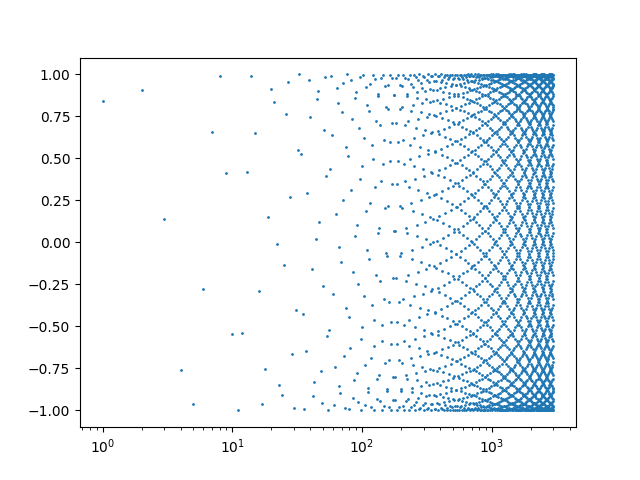# Integer sines

The following graph plots sin(1), sin(2), sin(3), etc. It is based on a graph I found on page 42 of Analysis by its History by Hairer and Wainer.Here’s the Python code that produced the plot.

```    import matplotlib.pyplot as plt
from numpy import arange, sin

x = arange(1, 3000)
plt.scatter(x, sin(x), s=1)
plt.xscale("log")
plt.savefig("int_sin.png")
```

Update: See this post where we look at a couple variations on this exercise.

## 2 thoughts on “Integer sines”

1. @Arnaldo: Nice page. It’s helpful to be able to interactively change the horizontal scale.